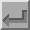Mirror Plane (m)The symbol above shows a mirror plane parallel to the plane of the screen. (Note that for non-orthogonal axes, the angle formed by the symbol is the same as the cell angle rather than 90°.) When present, this symbol is shown at the bottom left-hand corner of the space-group diagram. A fractional value next to the symbol indicates the height of the mirror above the XY plane. A mirror plane perpendicular to the c-axis and passing through the origin, i.e. the plane x,y,0, will have the corresponding symmetry operator x,y,-z. This plane has the written symbol "m".The symbol shown above corresponds to a mirror plane perpendicular to the plane of the screen with its normal perpendicular to the solid line. A mirror plane perpendicular to the b-axis and passing through the origin, i.e. the plane x,0,z, will have the corresponding symmetry operator x,-y,z.Back
 © Copyright 1995-2006.  Birkbeck College, University of London. Author(s): Jeremy Karl Cockcroft